@Team 2019-04-16T16:10:18.000000Z 字数 4513 阅读 2239

# 最后一届ImageNet冠军模型：SENet

叶虎

## 主体思路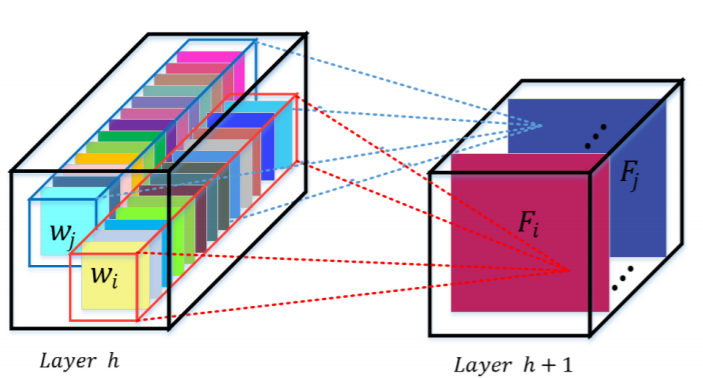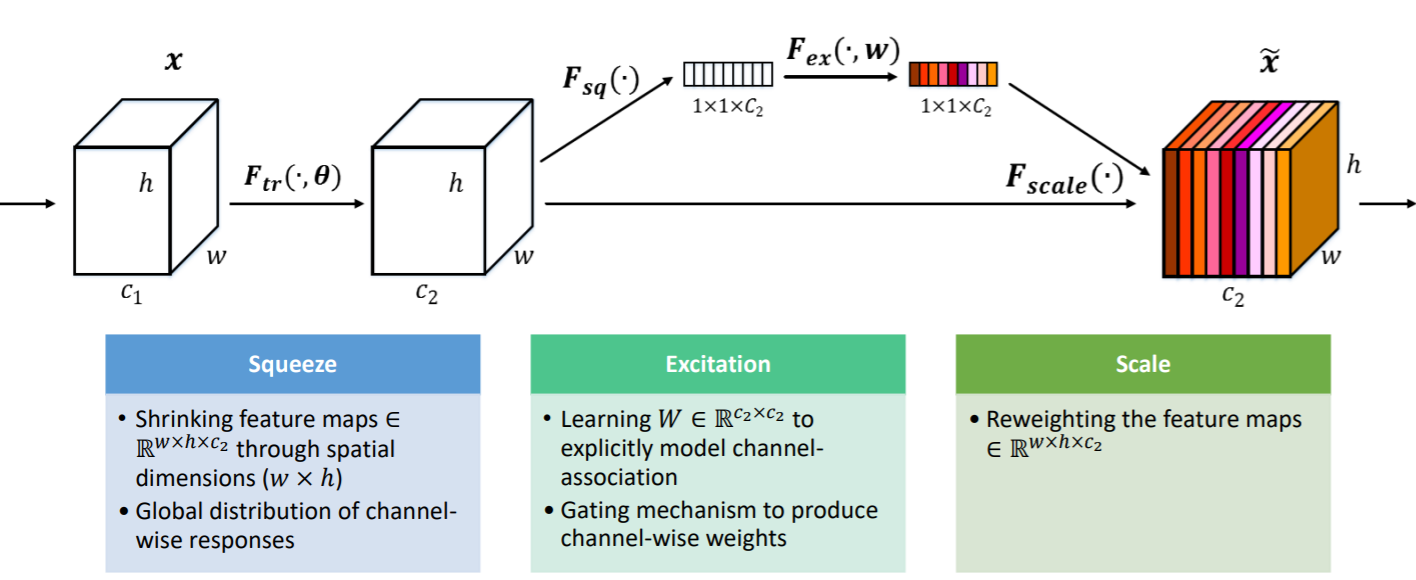SE模块首先对卷积得到的特征图进行Squeeze操作，得到channel级的全局特征，然后对全局特征进行Excitation操作，学习各个channel间的关系，也得到不同channel的权重，最后乘以原来的特征图得到最终特征。本质上，SE模块是在channel维度上做attention或者gating操作，这种注意力机制让模型可以更加关注信息量最大的channel特征，而抑制那些不重要的channel特征。另外一点是SE模块是通用的，这意味着其可以嵌入到现有的网络架构中。

## Squeeze-and-Excitation (SE) 模块

SE模块主要包括Squeeze和Excitation两个操作，可以适用于任何映射$F_{tr}: X\rightarrow U, X\in R^{H'\times W'\times C'}, U\in R^{H\times W\times C}$，以卷积为例，卷积核为$V=[v_1, v_2,...,v_C]$，其中$v_c$表示第c个卷积核。那么输出$U=[u_1, u_2,...,u_C]$

### Excitation操作

Sequeeze操作得到了全局描述特征，我们接下来需要另外一种运算来抓取channel之间的关系。这个操作需要满足两个准则：首先要灵活，它要可以学习到各个channel之间的非线性关系；第二点是学习的关系不是互斥的，因为这里允许多channel特征，而不是one-hot形式。基于此，这里采用sigmoid形式的gating机制：

## SE模块在Inception和ResNet上的应用

SE模块的灵活性在于它可以直接应用现有的网络结构中。这里以Inception和ResNet为例。对于Inception网络，没有残差结构，这里对整个Inception模块应用SE模块。对于ResNet，SE模块嵌入到残差结构中的残差学习分支中。具体如下图所示：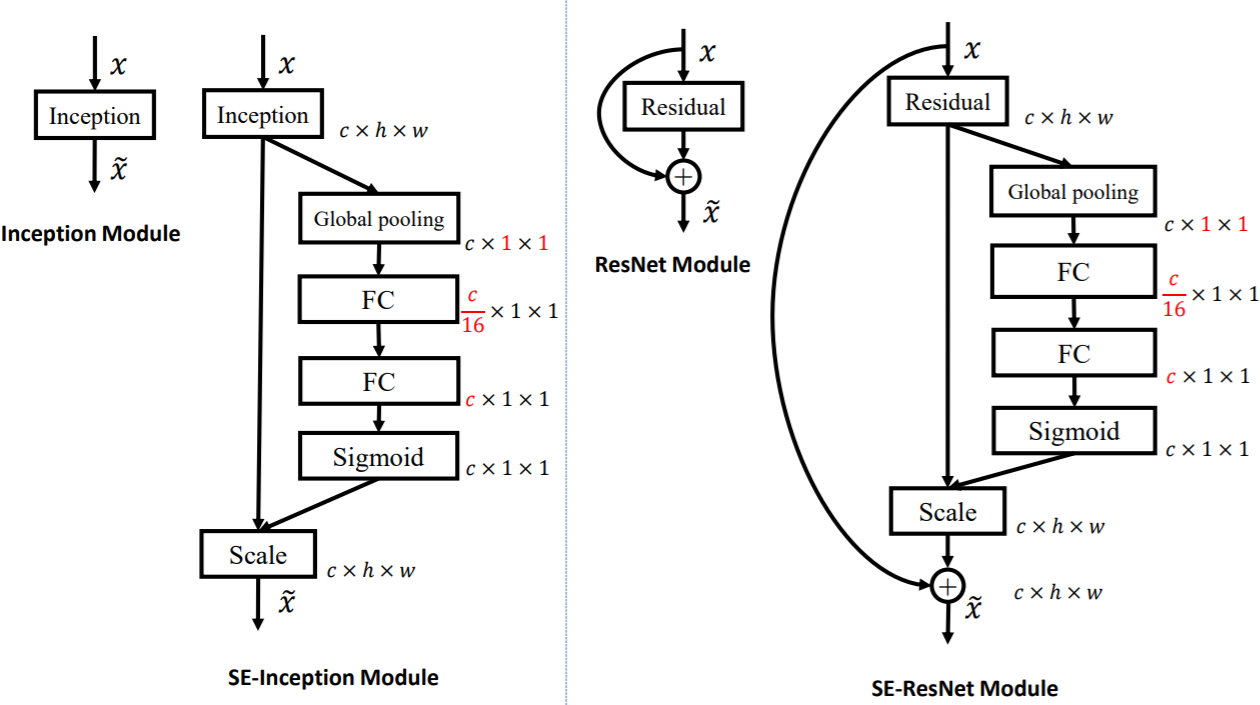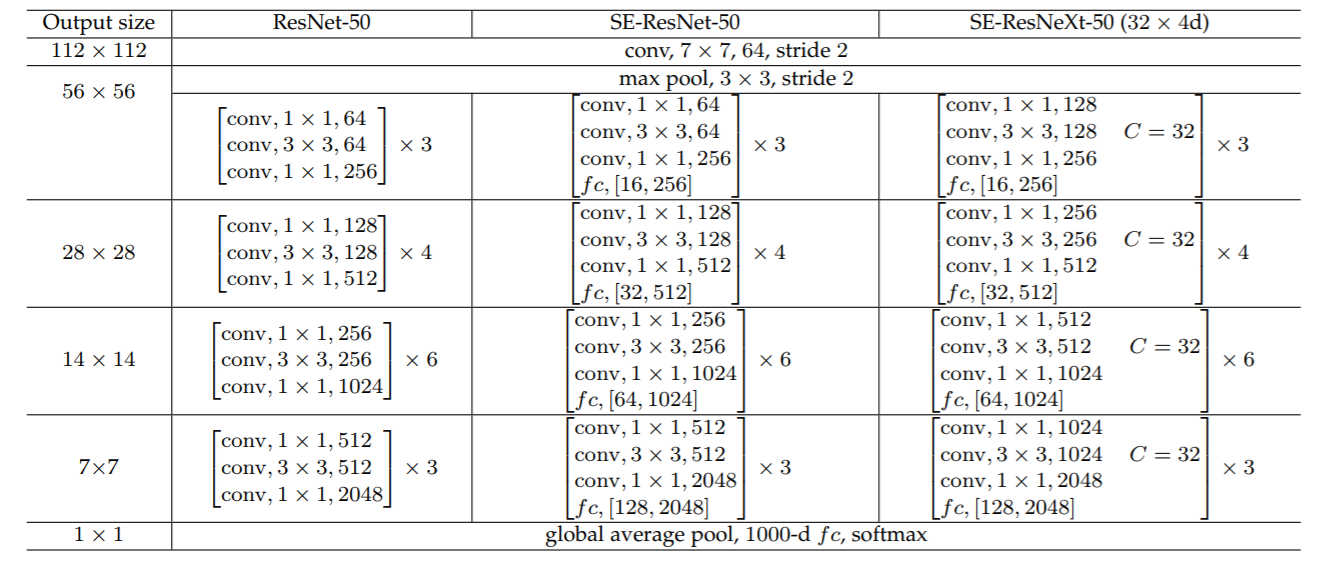## 模型效果

SE模块很容易嵌入到其它网络中，作者为了验证SE模块的作用，在其它流行网络如ResNet和VGG中引入SE模块，测试其在ImageNet上的效果，如下表所示：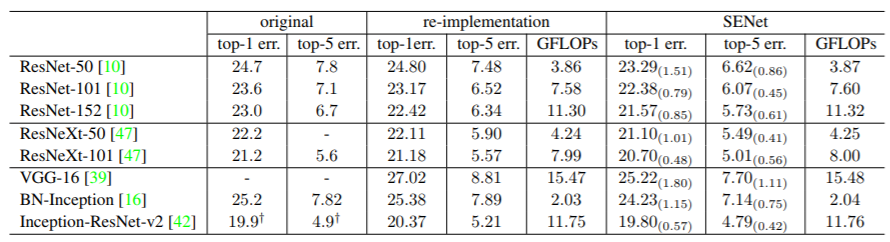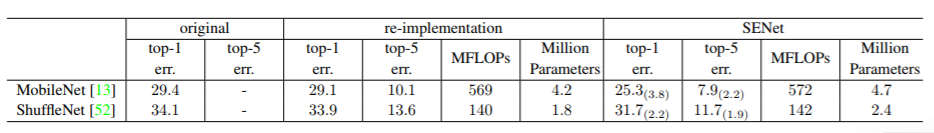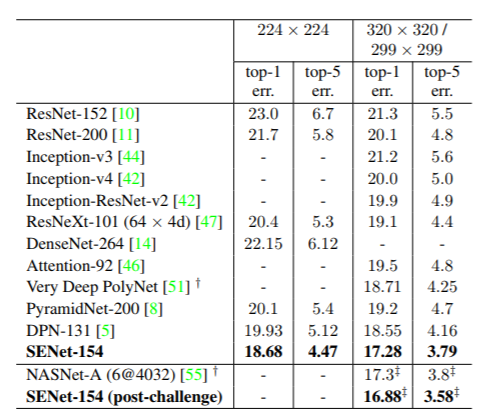## SE模块的实现

SE模块是非常简单的，实现起来也比较容易，这里给出PyTorch版本的实现（参考senet.pytorch）:

class SELayer(nn.Module):    def __init__(self, channel, reduction=16):        super(SELayer, self).__init__()        self.avg_pool = nn.AdaptiveAvgPool2d(1)        self.fc = nn.Sequential(            nn.Linear(channel, channel // reduction, bias=False),            nn.ReLU(inplace=True),            nn.Linear(channel // reduction, channel, bias=False),            nn.Sigmoid()        )    def forward(self, x):        b, c, _, _ = x.size()        y = self.avg_pool(x).view(b, c)        y = self.fc(y).view(b, c, 1, 1)        return x * y.expand_as(x)

    class SEBottleneck(nn.Module):        expansion = 4        def __init__(self, inplanes, planes, stride=1, downsample=None, reduction=16):            super(SEBottleneck, self).__init__()            self.conv1 = nn.Conv2d(inplanes, planes, kernel_size=1, bias=False)            self.bn1 = nn.BatchNorm2d(planes)            self.conv2 = nn.Conv2d(planes, planes, kernel_size=3, stride=stride,                                   padding=1, bias=False)            self.bn2 = nn.BatchNorm2d(planes)            self.conv3 = nn.Conv2d(planes, planes * 4, kernel_size=1, bias=False)            self.bn3 = nn.BatchNorm2d(planes * 4)            self.relu = nn.ReLU(inplace=True)            self.se = SELayer(planes * 4, reduction)            self.downsample = downsample            self.stride = stride        def forward(self, x):            residual = x            out = self.conv1(x)            out = self.bn1(out)            out = self.relu(out)            out = self.conv2(out)            out = self.bn2(out)            out = self.relu(out)            out = self.conv3(out)            out = self.bn3(out)            out = self.se(out)            if self.downsample is not None:                residual = self.downsample(x)            out += residual            out = self.relu(out)            return out

## 小结

SE模块主要为了提升模型对channel特征的敏感性，这个模块是轻量级的，而且可以应用在现有的网络结构中，只需要增加较少的计算量就可以带来性能的提升。另外最新的CVPR 2019有篇与SENet非常相似的网络SKNet（Selective Kernel Networks），SKNet主要是提升模型对感受野的自适应能力，感兴趣可以深入看一下。

## 参考文献• 私有
• 公开
• 删除